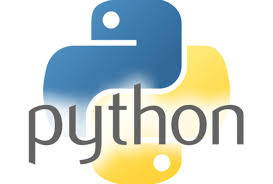11.What is the output of print str[2:5] if str = ‘Hello World!’?
It will print characters starting from 3rd to 5th. Output would be llo.

12.What is the output of print str[2:] if str = ‘Hello World!’?
It will print characters starting from 3rd character. Output would be llo World!.

13.What is the output of print str * 2 if str = ‘Hello World!’?
It will print string two times. Output would be Hello World!Hello World!.

14.What is the output of print str + “TEST” if str = ‘Hello World!’?
It will print concatenated string. Output would be Hello World!TEST.

15.What is the output of print list if list = [ ‘abcd’, 786 , 2.23, ‘john’, 70.2 ]?
It will print concatenated lists. Output would be [‘abcd’, 786, 2.23, ‘john’, 70.200000000000003, 123, ‘john’, 123, ‘john’].

16.What is the output of print list if list = [ ‘abcd’, 786 , 2.23, ‘john’, 70.2 ]?
It will print first element of the list. Output would be abcd.

17.What is the output of print list[1:3] if list = [ ‘abcd’, 786 , 2.23, ‘john’, 70.2 ]?
It will print elements starting from 2nd till 3rd. Output would be [786, 2.23].

18.What is the output of print list[2:] if list = [ ‘abcd’, 786 , 2.23, ‘john’, 70.2 ]?
It will print elements starting from 3rd element. Output would be [2.23, ‘john’, 70.200000000000003].

19.What is the output of print tinylist * 2 if tinylist = [123, ‘john’]?
It will print list two times. Output would be [123, ‘john’, 123, ‘john’].

20.What is the output of print list + tinylist * 2 if list = [ ‘abcd’, 786 , 2.23, ‘john’, 70.2 ] and tinylist = [123, ‘john’]?
It will print concatenated lists. Output would be [‘abcd’, 786, 2.23, ‘john’, 70.200000000000003, 123, ‘john’].